#### How to calculate covariance in Excel?

In this Excel tutorial, you will learn how to calculate covariance in Excel.

We can detect the existence of a correlation relationship by examining the covariance.

Covariance is the average of the products of the deviations of each data point pair.

Use covariance to define the relationship between two datasets.

• A positive value of covariance occurs when both examined characteristics are moving in the same direction.
• Negative covariance occurs when the increase in the value of one feature tends to decrease the value of the other.
• It is also possible that the covariance is zero. This means that the variables are not correlated with each other.

## Sample Covariance

To calculate sample covariance use the COVARIANCE.S Excel function.

### COVARIANCE.S syntax

=COVARIANCE.S(array1,array2)

In the given example sample covariance formula is =COVARIANCE.S(A2:A10,B2:B10)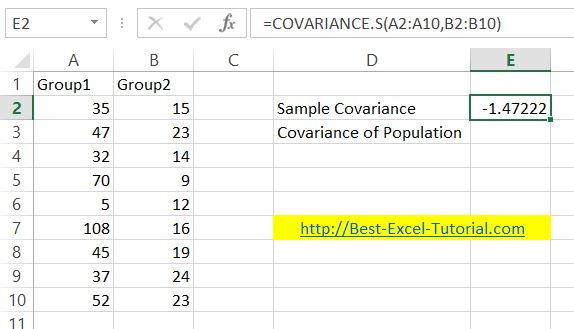## Population Covariance

To calculate population covariance use the COVARIANCE.P Excel function.

### COVARIANCE.P syntax

=COVARIANCE.S(array1,array2)

In the given example sample covariance formula is =COVARIANCE.P(A2:A10,B2:B10)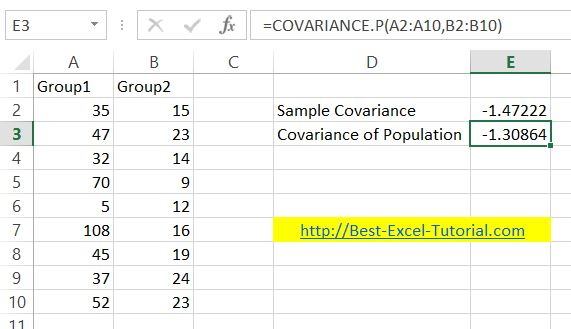In most cases, you will use sample covariance. Use covariance of population function only when it is specifically said that it is population covariance.

## Using the Data analysis tool

Using functions to enumerate the covariance is effective. I prefer to use the data analysis tool for covariance calculation. It is also a convenient way to calculate the covariance of a data table. Excel will prepare a covariance report based on the values ​​you provide.

`To use the data analysis tool, you must have the data analysis toolpak installed. Here you will find a course on how to install the data analysis toolpak in Excel.`

To calculate the covariance with the data analysis tool, first go to the Excel ribbon. Click the Data tab. On the right side you will find the Data Analysis button.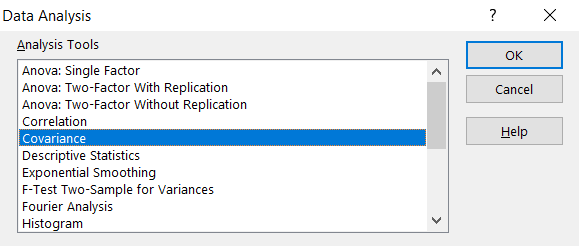A window will appear. Select Covariance from the menu.

In the next window, determine the input range. Covariance input is your entire data table. If you also select the row with headings, then check the Labels in the first row option.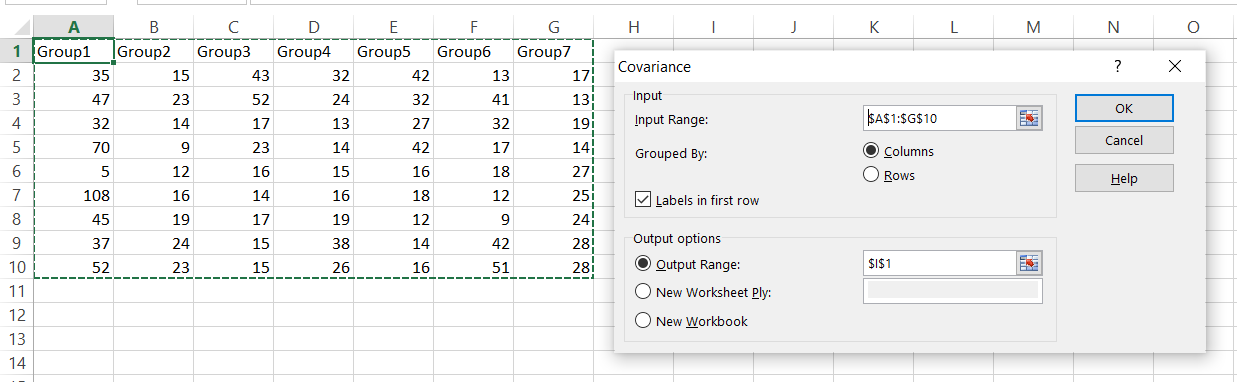Select the place where you want the result as the output range. If you have free space on the worksheet, enter the cell address. Otherwise, check New Worksheet Ply.

The covariance report has appeared in the position you selected as output in the previous window.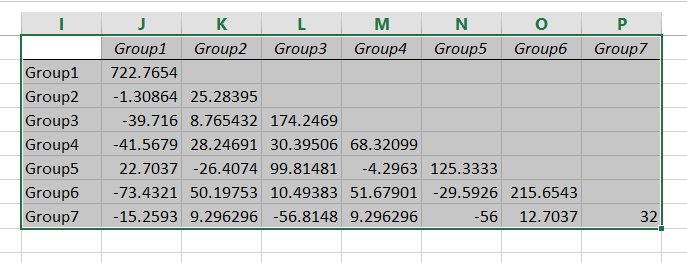It’s important to note that the covariance value alone does not give you a complete picture of the relationship between two variables. You should also consider other statistical measures such as the correlation coefficient, which measures the strength and direction of the linear relationship between two variables, and the coefficient of determination, which measures the proportion of the total variation in one variable that can be explained by the other variable.

## Using VBA code

To calculate covariance in Excel VBA, you can use the COVAR function, which is a built-in function in Excel that calculates the covariance between two sets of values.

Here’s an example of how to use the COVAR function in VBA to calculate the covariance between two sets of data:

`Sub CalculateCovariance()`
` Dim xValues As Range, yValues As Range`
` Dim covariance As Double`

` ' Set the range of cells containing the X values`
` Set xValues = Range("A2:A6")`

` ' Set the range of cells containing the Y values`
` Set yValues = Range("B2:B6")`

` ' Calculate the covariance using the COVAR function`
` covariance = Application.WorksheetFunction.Covar(xValues, yValues)`

` ' Output the result`
` Range("C2").Value = "Covariance:"`
` Range("D2").Value = covariance`
`End Sub`

In this example, we first define two ranges of cells for the X values and Y values, respectively. We then use the COVAR function, which takes the two ranges as input arguments and returns the covariance between them.